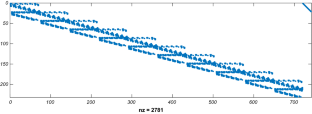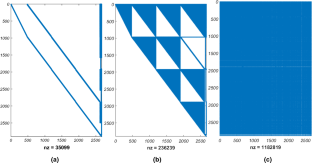# Efficient Graph-Theoretical Force Method: Wedge-Shaped Finite Element

## Abstract

Formation of a suitable null basis (static basis) for equilibrium matrix is the main part of finite element analysis via force method. For an optimal analysis, the selected null basis matrices should be sparse, well-structured, e.g., banded, and well-conditioned flexibility matrices. In this paper, an efficient method is developed for the formation of null bases of wedge element, corresponding to highly sparse and banded flexibility matrices. This is achieved by associating special graphs with the FEM and selecting appropriate subgraphs and forming the self-equilibrating systems on these subgraphs. The efficiency of the presented method is illustrated through three examples.

This is a preview of subscription content, access via your institution.Fig. 1Fig. 2Fig. 3Fig. 4Fig. 5Fig. 6Fig. 7Fig. 8Fig. 9Fig. 10Fig. 11Fig. 12Fig. 13Fig. 14Fig. 15Fig. 16Fig. 17Fig. 18Fig. 19Fig. 20Fig. 21Fig. 22

## References

1. Argyris JH, Kelsey S (1960) Energy theorems and structural analysis. Butterworth, London

2. Coleman TF, Pothen A (1986) The null space problem I; complexity. SIAM J Alg Disc Methods 7:527–537

3. Coleman TF, Pothen A (1987) The null space problem II; algorithms. SIAM J Alg Disc Methods 8:544–561

4. Denke PH (1962) A general digital computer analysis of statically indeterminate structures. NASA-TD-D-1666

5. Heath MT, Plemmons R, Ward J (1984) Sparse orthogonal schemes for structural optimization using the force method. SIAM J Sci Stat Comput 5:514–532

6. Henderson JCC, Maunder EAW (1969) A problem in applied topology: on the selection of cycles for the flexibility analysis of skeletal structures. J Inst Math Appl 5(2):254–269

7. Kaneko I, Lawo M, Thierauf G (1982) On computational procedures for the force methods. Int J Numer Methods Eng 18:1469–1495

8. Kaveh A (1974) Application of topology and matroid theory to the analysis of structures. PhD thesis, London University, Imperial College

9. Kaveh A (1979) A combinatorial optimization problem: optimal generalized cycle bases. Comput Methods Appl Mech Eng 20:39–52

10. Kaveh A (1992) Recent developments in the force method of structural analysis. Appl Mech Rev 45:401–418

11. Kaveh A (2004) Structural mechanics: graph and matrix methods, 3rd edn. RSP, Exeter

12. Kaveh A (2006) Optimal structural analysis, 2nd edn. Research Studies Press, Letchworth

13. Kaveh A (2014) Computational structural analysis and finite element methods. Springer, New York

14. Kaveh A, Massoudi MS (2015) Efficient finite element analysis using graph-theoretical force method; rectangular plane stress and plane strain Lagrange family elements. Appl Math Comput 266:72–95

15. Kaveh A, Naseri Nasab E (2010) A new four-node quadrilateral plate bending element for highly sparse and banded flexibility matrices. Acta Mech 209:295–309

16. Kaveh A, Tolou Kian MJ (2012) Efficient finite element analysis using graph-theoretical force method with brick elements. Finite Elem Anal Des 54:1–15

17. Kaveh A, Massoudi MS, Massoudi MJ (2013) Efficient finite element analysis using graph-theoretical force method; rectangular plane stress and plane strain serendipity family elements. Period Polytech Civ Eng 58:1–20

18. Kazemzadeh Azad S, Hasançebi O (2015) Discrete sizing optimization of steel trusses under multiple displacement constraints and load cases using guided stochastic search technique. Struct Multidisc Optim 52:383–404

19. Patnaik SN (1973) An integrated force method for discrete analysis. Int J Numer Methods Eng 6:237–251

20. Pothen A (1989) Sparse null basis computation in structural optimization. Numer Math 55:501–519

21. Przemieniecki JS (1968) Theory of matrix structural analysis. McGraw-Hill, New York

22. Robinson J (1973) Integrated theory of finite element methods. Wiley, New York

23. Soyer E, Topçu A (2001) Sparse self-stress matrices for the finite element force method. Int J Numer Methods Eng 50:2175–2194

24. Tohidi SS, Khaki Sedigh A, Buzorgnia D (2016) Fault tolerant control design using adaptive control allocation based on the pseudo inverse along the null space. Int J Robust Nonlinear Control 26(16):3541–3557

25. Wang H, Li E, Li G (2013) A parallel reanalysis method based on approximate inverse matrix for complex engineering problems. J Mech Des 135(8):081001

Download references

## Author information

Authors

### Corresponding author

Correspondence to M. S. Massoudi.

## Rights and permissions

Reprints and Permissions